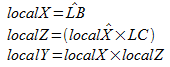Thursday, April 22, 2010

Tangent-space basis vectors: Don't assume your texture projection is orthogonal

How do you generate the tangent vectors, which represent which way the texture axes on a textured triangle, are facing?

Hitting up Google tends to produce articles like this one, or maybe even that exact one. I've seen others linked too, the basic formulae tend to be the same. Have you looked at what you're pasting into your code though? Have you noticed that you're using the T coordinates to calculate the S vector, and vice versa? Well, you can look at the underlying math, and you'll find that it's because that's what happens when you assume the normal, S vector, and T vectors form an orthonormal matrix and attempt to invert it, in a sense you're not really using the S and T vectors but rather vectors perpendicular to them.

But that's fine, right? I mean, this is an orthogonal matrix, and they are perpendicular to each other, right? Well, does your texture project on to the triangle with the texture axes at right angles to each other, like a grid?... Not always? Well, you might have a problem then!

Well, what do we know? First, translating the vertex positions will not affect the axial directions. Second, scrolling the texture will not affect the axial directions.

So, for triangle (A,B,C), with coordinates (x,y,z,t), we can create a new triangle (LA,LB,LC) and the directions will be the same:

We also know that both axis directions are on the same plane as the points, so to resolve that, we can to convert this into a local coordinate system and force one axis to zero.Now we need triangle (Origin, PLB, PLC) in this local coordinate space. We know PLB[y] is zero since LB was used as the X axis.Now we can solve this. Remember that PLB[y] is zero, so...Do this for both axes and you have your correct texture axis vectors, regardless of the texture projection. You can then multiply the results by your tangent-space normalmap, normalize the result, and have a proper world-space surface normal.

As always, the source code spoilers:

terVec3 lb = ti->points - ti->points;
terVec3 lc = ti->points - ti->points;
terVec2 lbt = ti->texCoords - ti->texCoords;
terVec2 lct = ti->texCoords - ti->texCoords;

// Generate local space for the triangle plane
terVec3 localX = lb.Normalize2();
terVec3 localZ = lb.Cross(lc).Normalize2();
terVec3 localY = localX.Cross(localZ).Normalize2();

// Determine X/Y vectors in local space
float plbx = lb.DotProduct(localX);
terVec2 plc = terVec2(lc.DotProduct(localX), lc.DotProduct(localY));

terVec2 tsvS, tsvT;

tsvS = lbt / plbx;
tsvS = (lct - tsvS*plc) / plc;
tsvT = lbt / plbx;
tsvT = (lct - tsvT*plc) / plc;

ti->svec = (localX*tsvS + localY*tsvS).Normalize2();
ti->tvec = (localX*tsvT + localY*tsvT).Normalize2();

There's an additional special case to be aware of: Mirroring.

Mirroring across an edge can cause wild changes in a vector's direction, possibly even degenerating it. There isn't a clear-cut solution to these, but you can work around the problem by snapping the vector to the normal, effectively cancelling it out on the mirroring edge.

Personally, I check the angle between the two vectors, and if they're more than 90 degrees apart, I cancel them, otherwise I merge them.